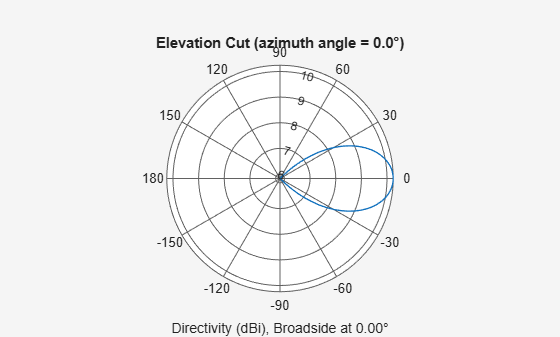Documentation

# patternElevation

System object: phased.PartitionedArray
Package: phased

Plot partitioned array directivity or pattern versus elevation

## Syntax

```patternElevation(sArray,FREQ) patternElevation(sArray,FREQ,AZ) patternElevation(sArray,FREQ,AZ,Name,Value) PAT = patternElevation(___) ```

## Description

`patternElevation(sArray,FREQ)` plots the 2-D array directivity pattern versus elevation (in dBi) for the array `sArray` at zero degrees azimuth angle. When `AZ` is a vector, multiple overlaid plots are created. The argument `FREQ` specifies the operating frequency.

`patternElevation(sArray,FREQ,AZ)`, in addition, plots the 2-D element directivity pattern versus elevation (in dBi) at the azimuth angle specified by `AZ`. When `AZ` is a vector, multiple overlaid plots are created.

`patternElevation(sArray,FREQ,AZ,Name,Value)` plots the array pattern with additional options specified by one or more `Name,Value` pair arguments.

`PAT = patternElevation(___)` returns the array pattern. `PAT` is a matrix whose entries represent the pattern at corresponding sampling points specified by the `'Elevation'` parameter and the `AZ` input argument.

## Input Arguments

expand all

Partitioned array, specified as a `phased.PartitionedArray` System object.

Example: `sArray= phased.PartitionedArray;`

Frequency for computing directivity and pattern, specified as a positive scalar. Frequency units are in hertz.

• For an antenna or microphone element, `FREQ` must lie within the range of values specified by the `FrequencyRange` or the `FrequencyVector` property of the element. Otherwise, the element produces no response and the directivity is returned as `–Inf`. Most elements use the `FrequencyRange` property except for `phased.CustomAntennaElement` and `phased.CustomMicrophoneElement`, which use the `FrequencyVector` property.

• For an array of elements, `FREQ` must lie within the frequency range of the elements that make up the array. Otherwise, the array produces no response and the directivity is returned as `–Inf`.

Example: `1e8`

Data Types: `double`

Azimuth angles for computing sensor or array directivities and patterns, specified as a 1-by-N real-valued row vector where N is the number of desired azimuth directions. Angle units are in degrees. The azimuth angle must lie between –180° and 180°.

The azimuth angle is the angle between the x-axis and the projection of the direction vector onto the xy plane. This angle is positive when measured from the x-axis toward the y-axis.

Example: `[0,10,20]`

Data Types: `double`

### Name-Value Pair Arguments

Specify optional comma-separated pairs of `Name,Value` arguments. `Name` is the argument name and `Value` is the corresponding value. `Name` must appear inside quotes. You can specify several name and value pair arguments in any order as `Name1,Value1,...,NameN,ValueN`.

Displayed pattern type, specified as the comma-separated pair consisting of `'Type'` and one of

• `'directivity'` — directivity pattern measured in dBi.

• `'efield'` — field pattern of the sensor or array. For acoustic sensors, the displayed pattern is for the scalar sound field.

• `'power'` — power pattern of the sensor or array defined as the square of the field pattern.

• `'powerdb'` — power pattern converted to dB.

Example: `'powerdb'`

Data Types: `char`

Signal propagation speed, specified as the comma-separated pair consisting of `'PropagationSpeed'` and a positive scalar in meters per second.

Example: `'PropagationSpeed',physconst('LightSpeed')`

Data Types: `double`

Subarray weights, specified as the comma-separated pair consisting of `'Weights'` and an M-by-1 complex-valued column vector. Subarray weights are applied to the subarrays of the array to produce array steering, tapering, or both. The dimension M is the number of subarrays in the array.

Example: `'Weights',ones(10,1)`

Data Types: `double`
Complex Number Support: Yes

Subarray steering angle, specified as the comma-separated pair consisting of `'SteerAngle'` and a scalar or a 2-by-1 column vector.

If `'SteerAngle'` is a 2-by-1 column vector, it has the form `[azimuth; elevation]`. The azimuth angle must be between –180° and 180°, inclusive. The elevation angle must be between –90° and 90°, inclusive.

If `'SteerAngle'` is a scalar, it specifies the azimuth angle only. In this case, the elevation angle is assumed to be 0.

This option applies only when the `'SubarraySteering'` property of the System object is set to `'Phase'` or `'Time'`.

Example: `'SteerAngle',[20;30]`

Data Types: `double`

Subarray element weights, specified as complex-valued NSE-by-N matrix or 1-by-N cell array. Weights are applied to the individual elements within a subarray. Subarrays can have different dimensions and sizes.

If `ElementWeights` is a complex-valued NSE-by-N matrix, NSE is the number of elements in the largest subarray and N is the number of subarrays. Each column of the matrix specifies the weights for the corresponding subarray. Only the first K entries in each column are applied as weights where K is the number of elements in the corresponding subarray.

If `ElementWeights` is a 1-by-N cell array. Each cell contains a complex-valued column vector of weights for the corresponding subarray. The column vectors have lengths equal to the number of elements in the corresponding subarray.

#### Dependencies

To enable this name-value pair, set the `SubarraySteering` property of the array to `'Custom'`.

Data Types: `double`
Complex Number Support: Yes

Elevation angles, specified as the comma-separated pair consisting of `'Elevation'` and a 1-by-P real-valued row vector. Elevation angles define where the array pattern is calculated.

Example: `'Elevation',[-90:2:90]`

Data Types: `double`

## Output Arguments

expand all

Array directivity or pattern, returned as an L-by-N real-valued matrix. The dimension L is the number of elevation angles determined by the `'Elevation'` name-value pair argument. The dimension N is the number of azimuth angles determined by the `AZ` argument.

## Examples

expand all

Convert a 2-by-6 URA of isotropic antenna elements into a 1-by-3 partitioned array so that each subarray of the partitioned array is a 2-by-2 URA. Assume that the frequency response of the elements lies between 1 and 6 GHz. The elements are spaced one-half wavelength apart corresponding to the highest frequency of the element response. Plot the directivity for elevation angles from -45 to 45 degrees. For partitioned arrays, weights are applied to the subarrays instead of the elements.

Create partitioned array

```fmin = 1e9; fmax = 6e9; c = physconst('LightSpeed'); lam = c/fmax; sIso = phased.IsotropicAntennaElement(... 'FrequencyRange',[fmin,fmax],... 'BackBaffled',false); sURA = phased.URA('Element',sIso,'Size',[2,6],... 'ElementSpacing',[lam/2,lam/2]); subarraymap = [[1,1,1,1,0,0,0,0,0,0,0,0];... [0,0,0,0,1,1,1,1,0,0,0,0];... [0,0,0,0,0,0,0,0,1,1,1,1]]; sPA = phased.PartitionedArray('Array',sURA,... 'SubarraySelection',subarraymap);```

Plot elevation directivity pattern

Plot the response of the array at 5 GHz

```fc = 5e9; wts = [0.862,1.23,0.862]'; azimangle = 0; patternElevation(sPA,fc,azimangle,... 'Type','directivity',... 'PropagationSpeed',physconst('LightSpeed'),... 'Elevation',[-45:45],... 'Weights',wts)```